# NCERT Exemplar Class 9 Science Solutions for Chapter 4 - Structure of the Atom

## NCERT Exemplar Solutions Class 9 Science Chapter 4 – Free PDF Download

NCERT Exemplar Solutions for Class 9 Science Chapter 4 Structure of Atom help students to learn the concepts thoroughly discussed in CBSE Class 9 Structure of Atom. Referring to NCERT Exemplar aids in laying a strong foundation for students’ future studies. Topics involved here are the basics and are helpful throughout their life. The PDF format of solutions is free to download and can be used by students while answering the questions from the NCERT Exemplar textbook.

NCERT Exemplar Solutions Class 9 Science provide you with MCQs, Atomic Structure short answer questions and long answers that will help you analyse the possible types of questions that may appear in Class 9 CBSE examinations and competitive examinations. Instead of searching for the best reference book, students can directly access the solutions from BYJU’S. The answers for each and every chapter are designed by the subject experts with utmost care to help students grasp the important concepts.

### Download the PDF the of NCERT Exemplar for Class 9 Science Chapter 4 – Structure of the Atom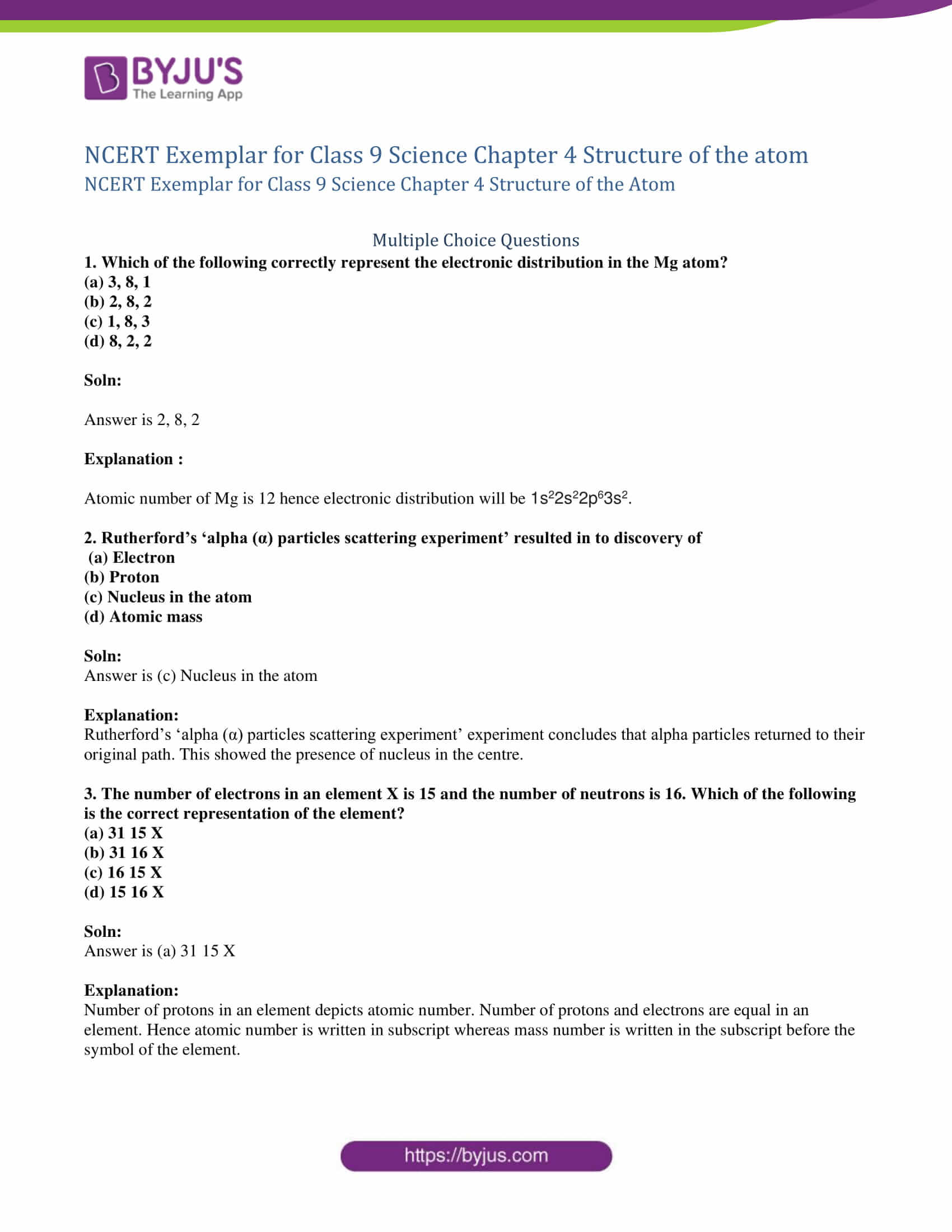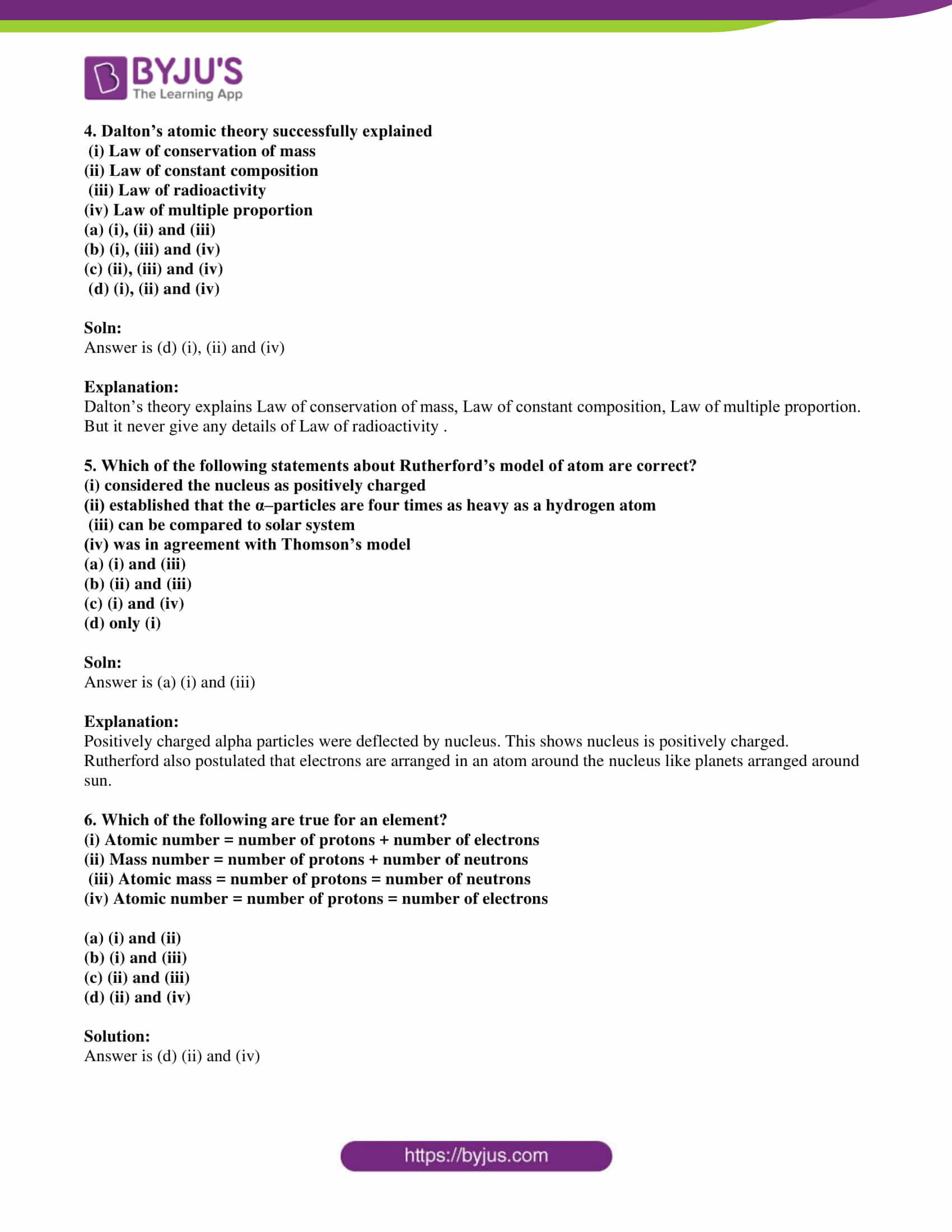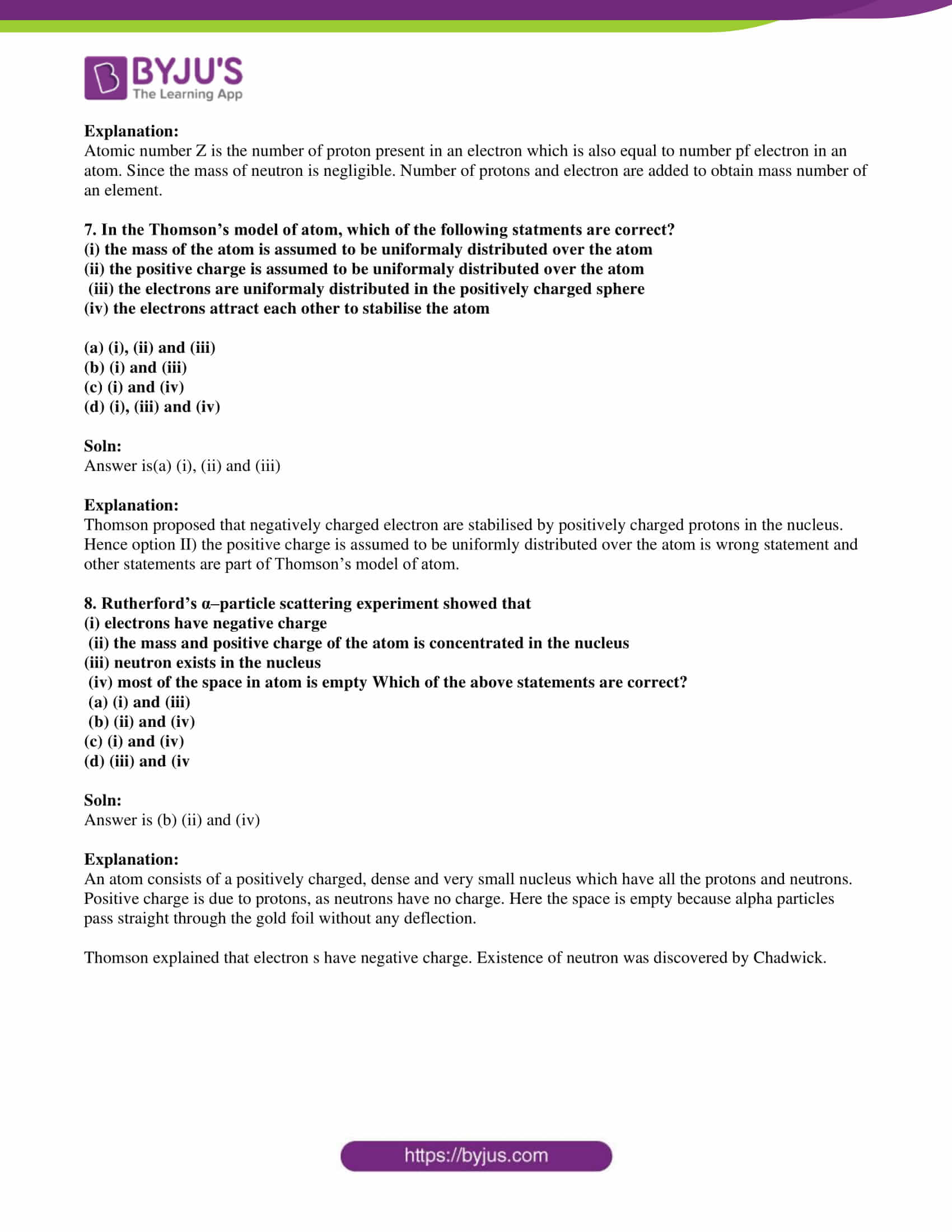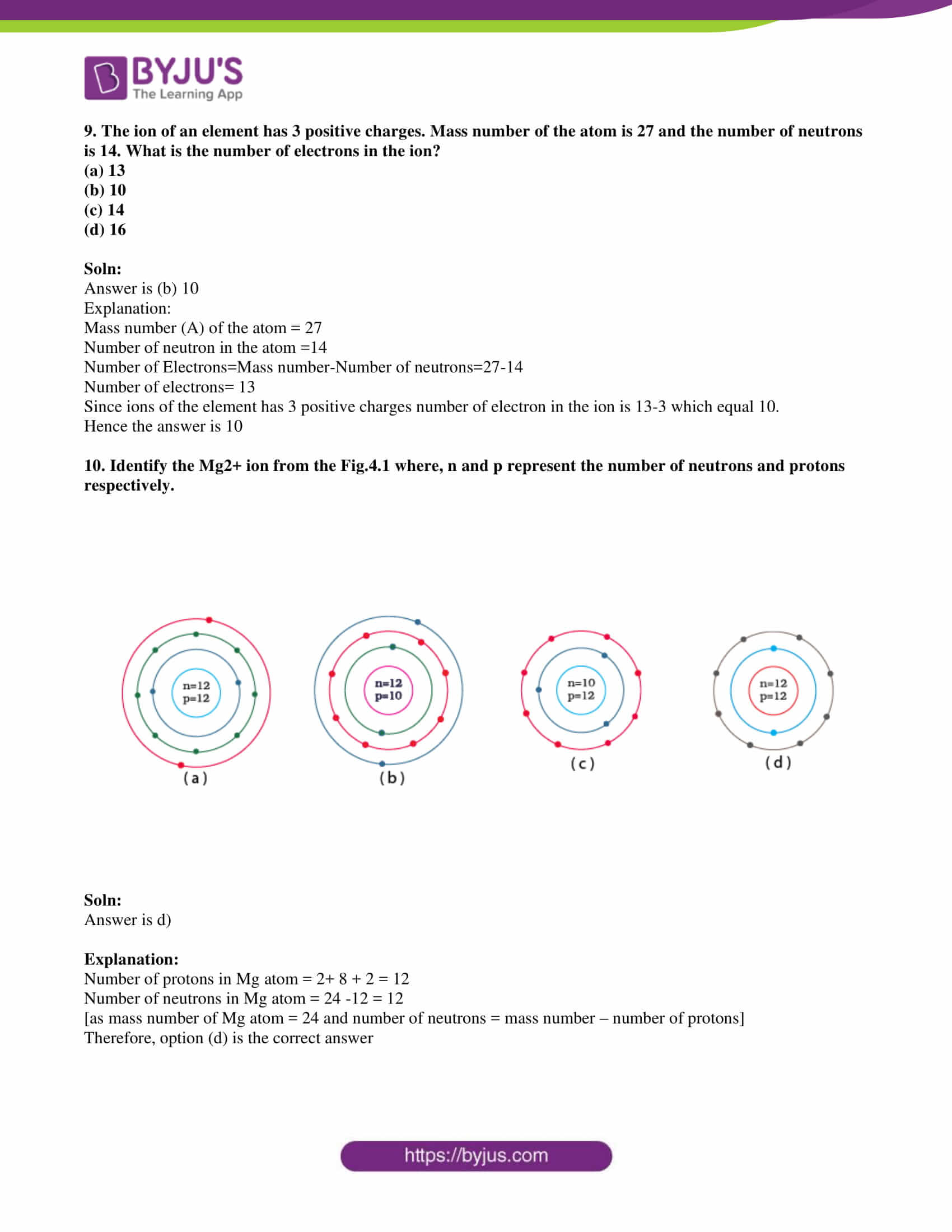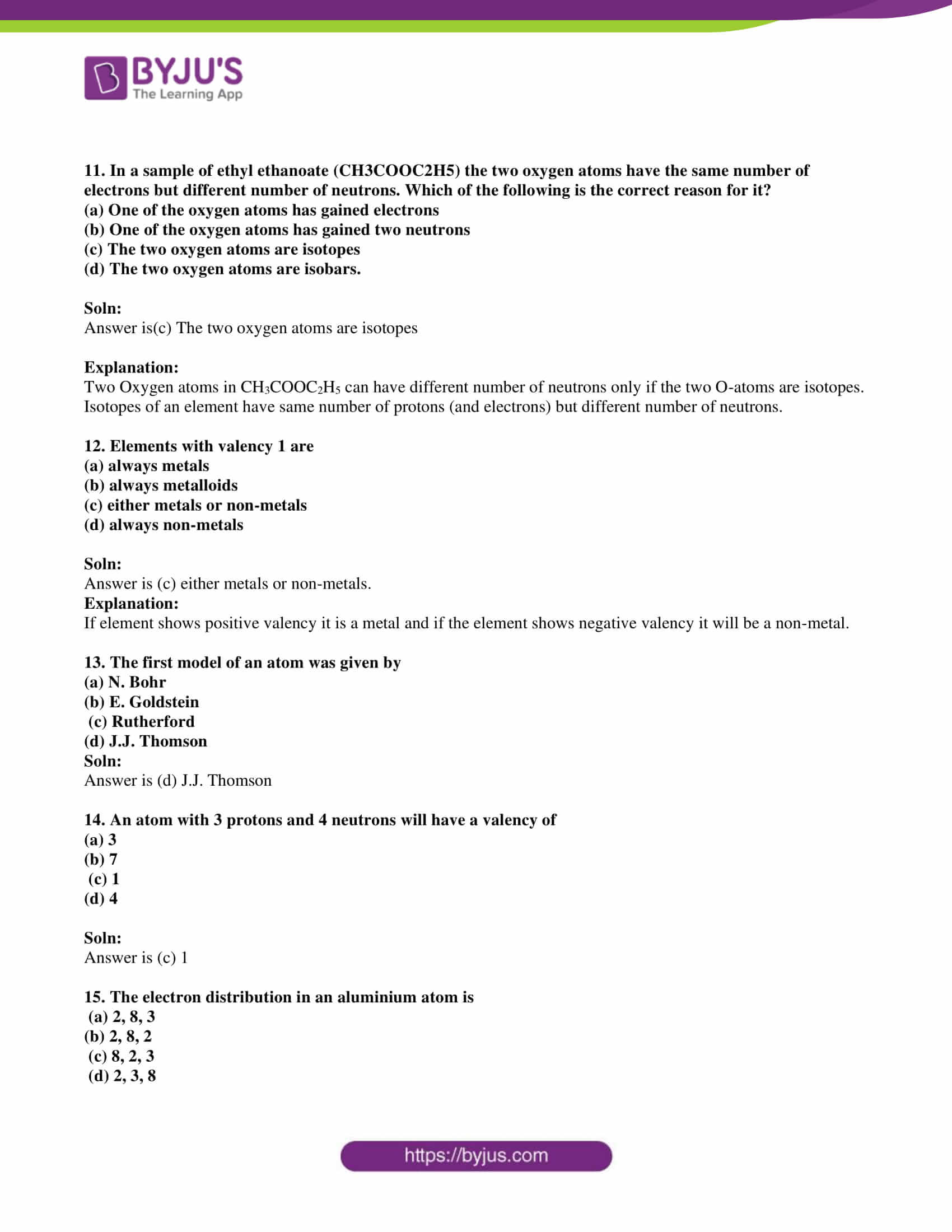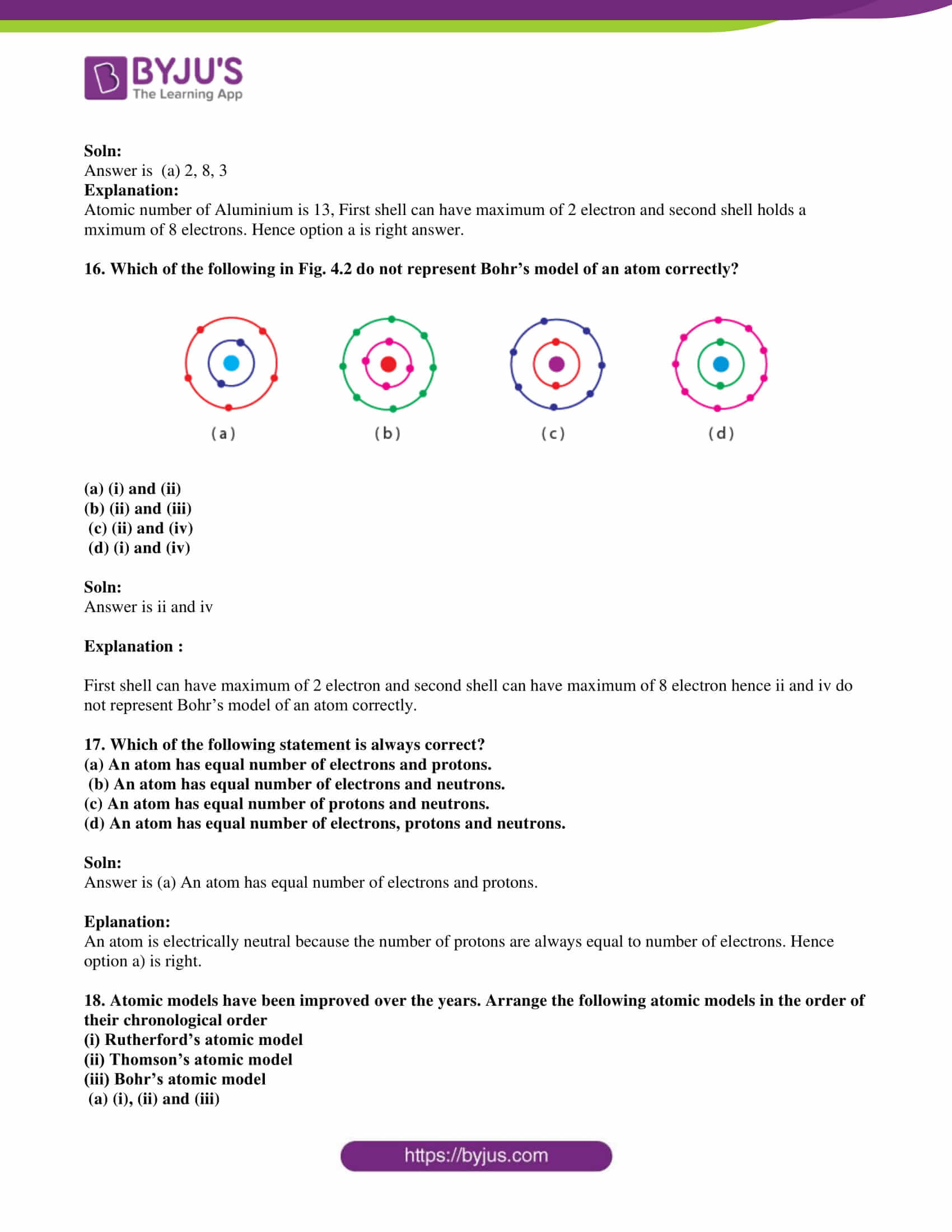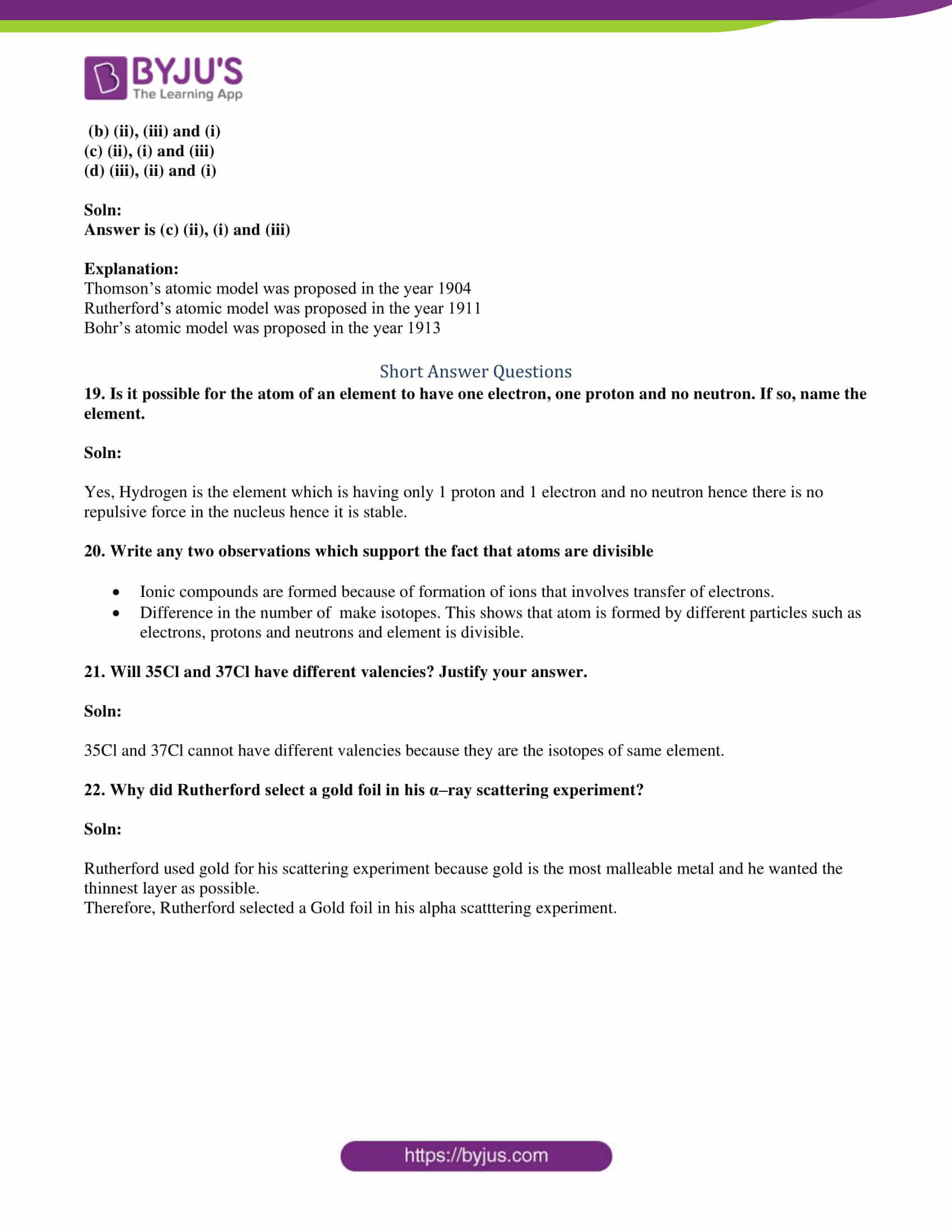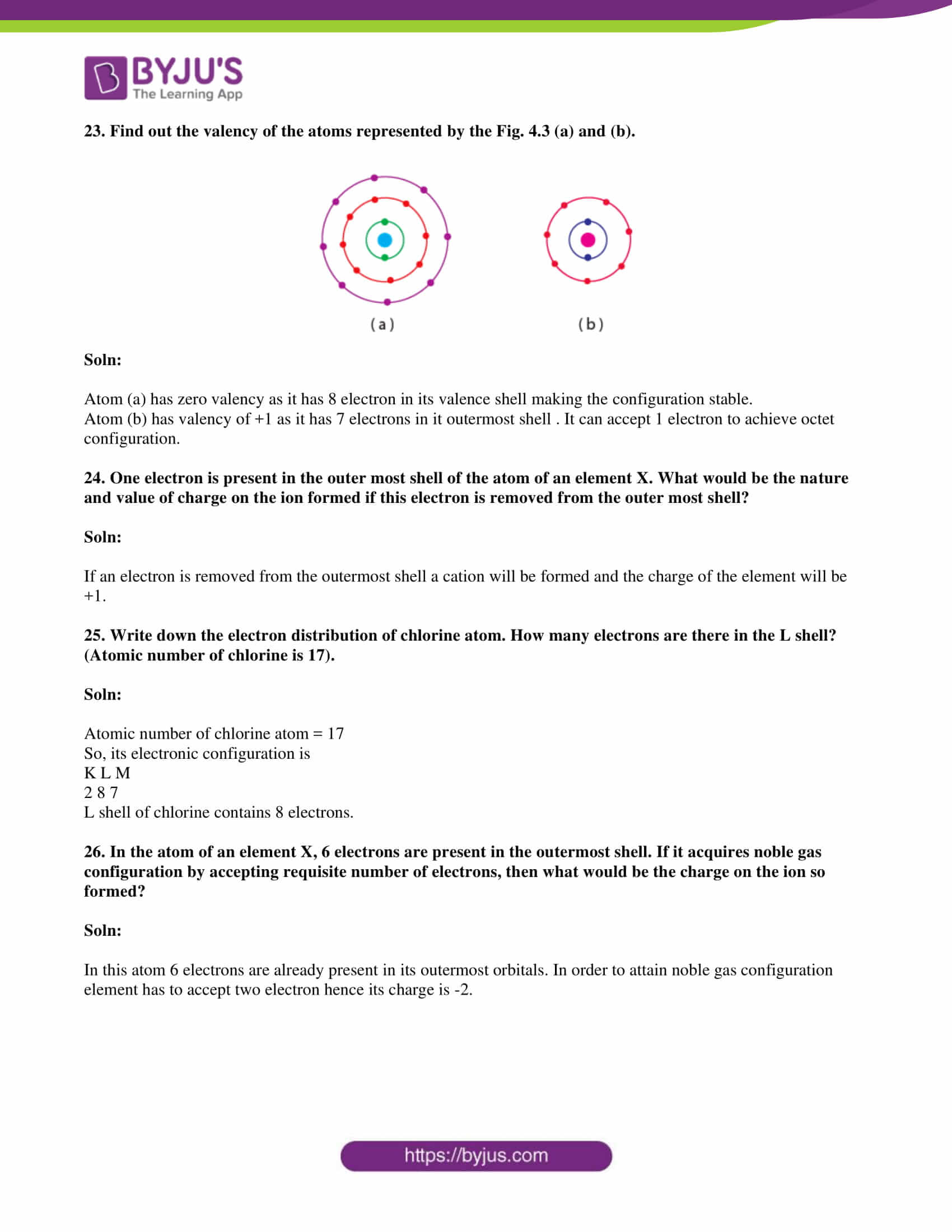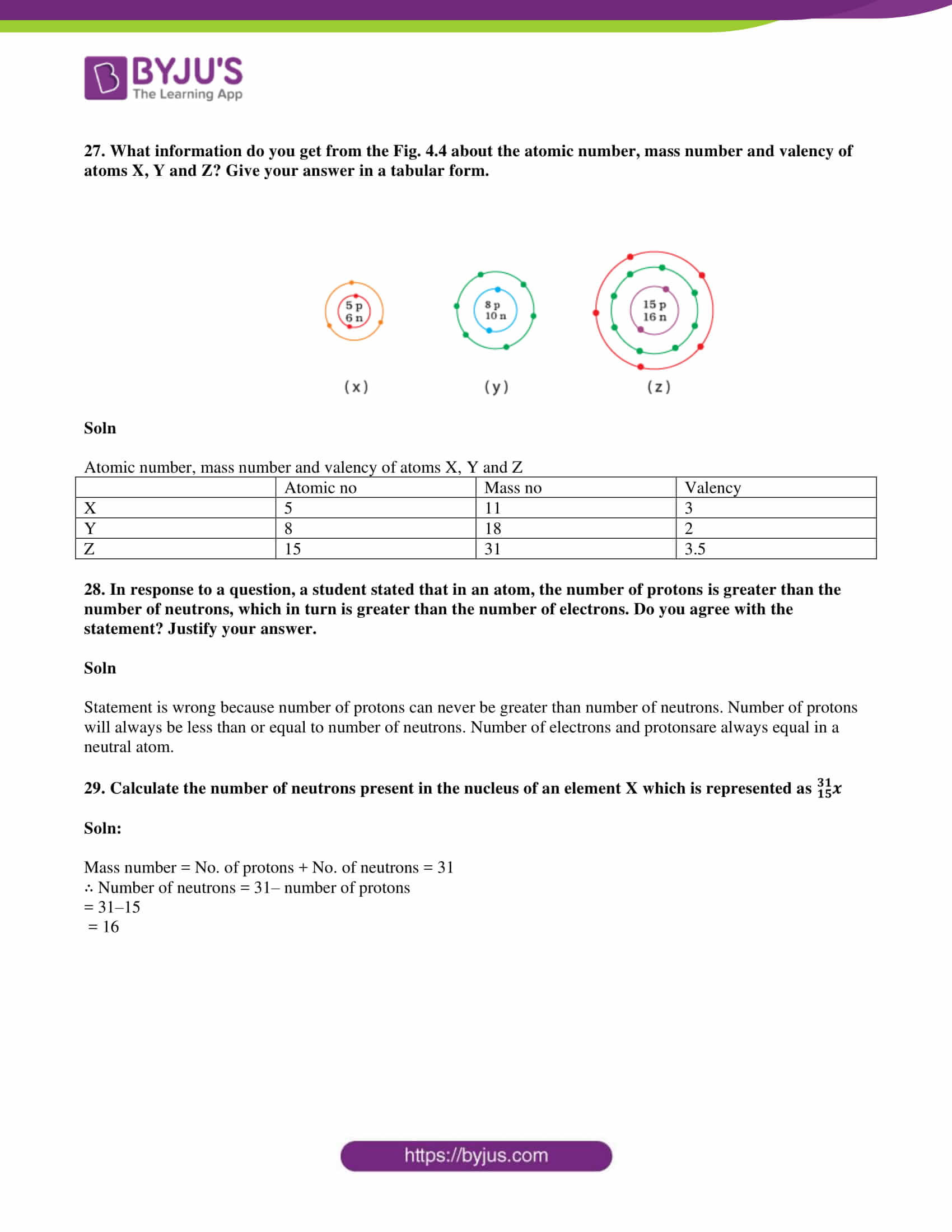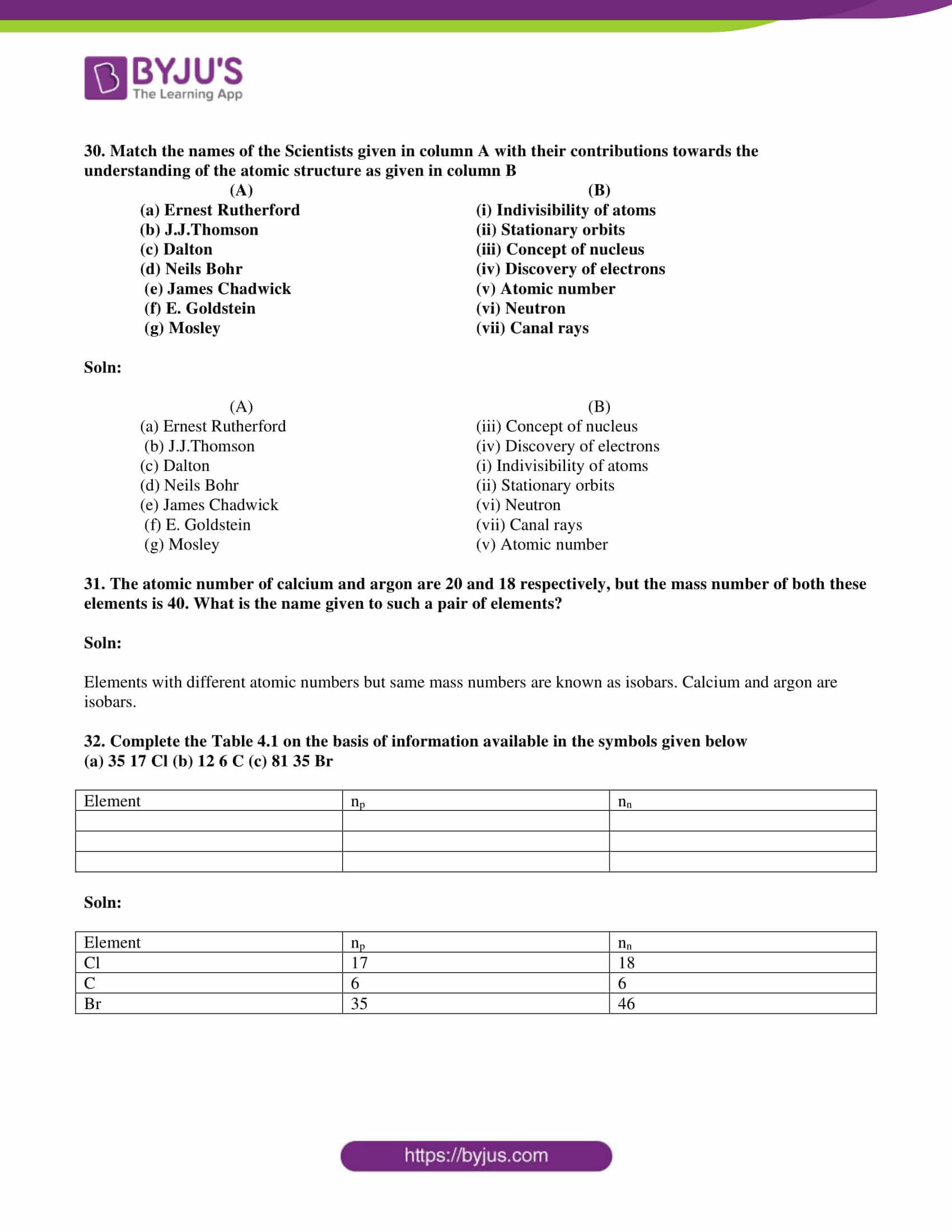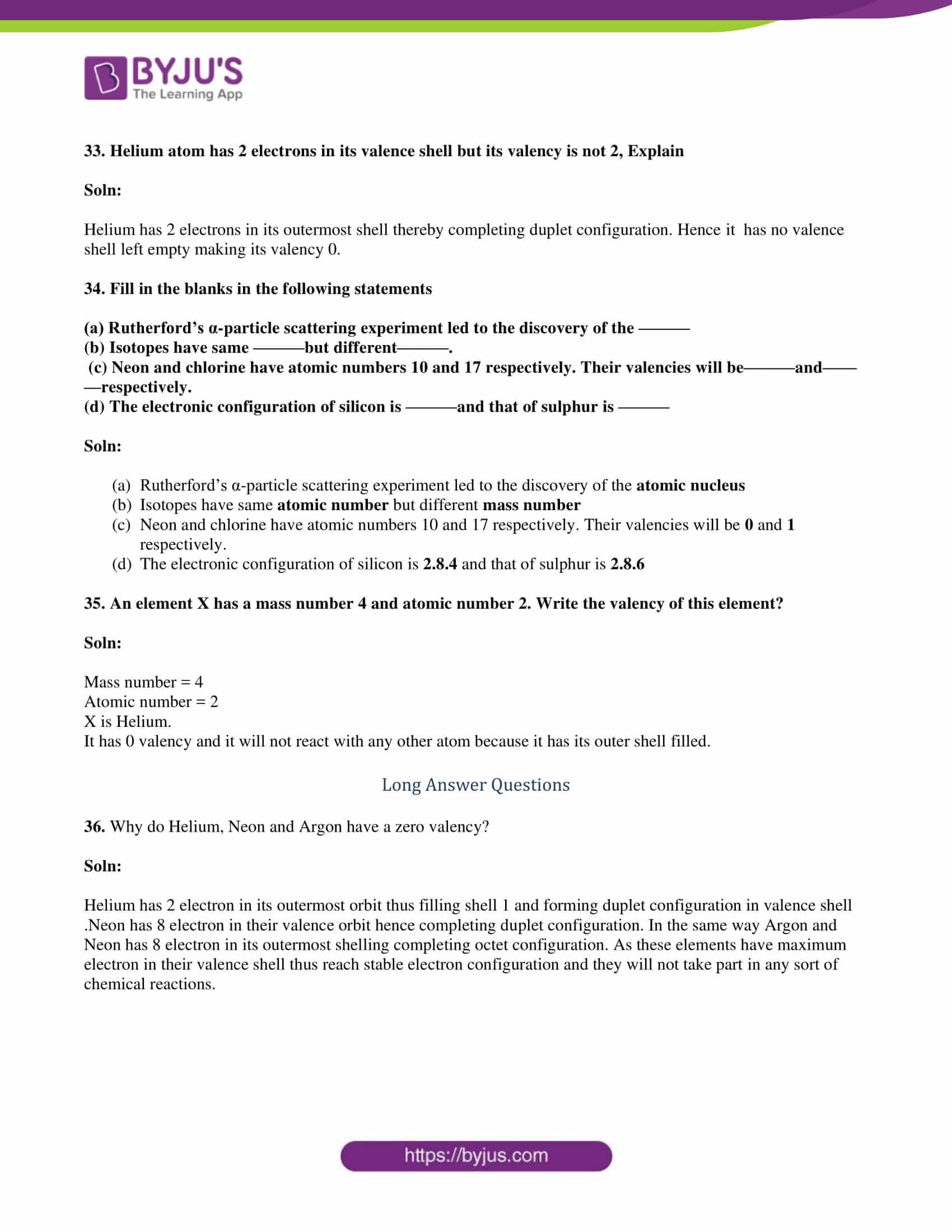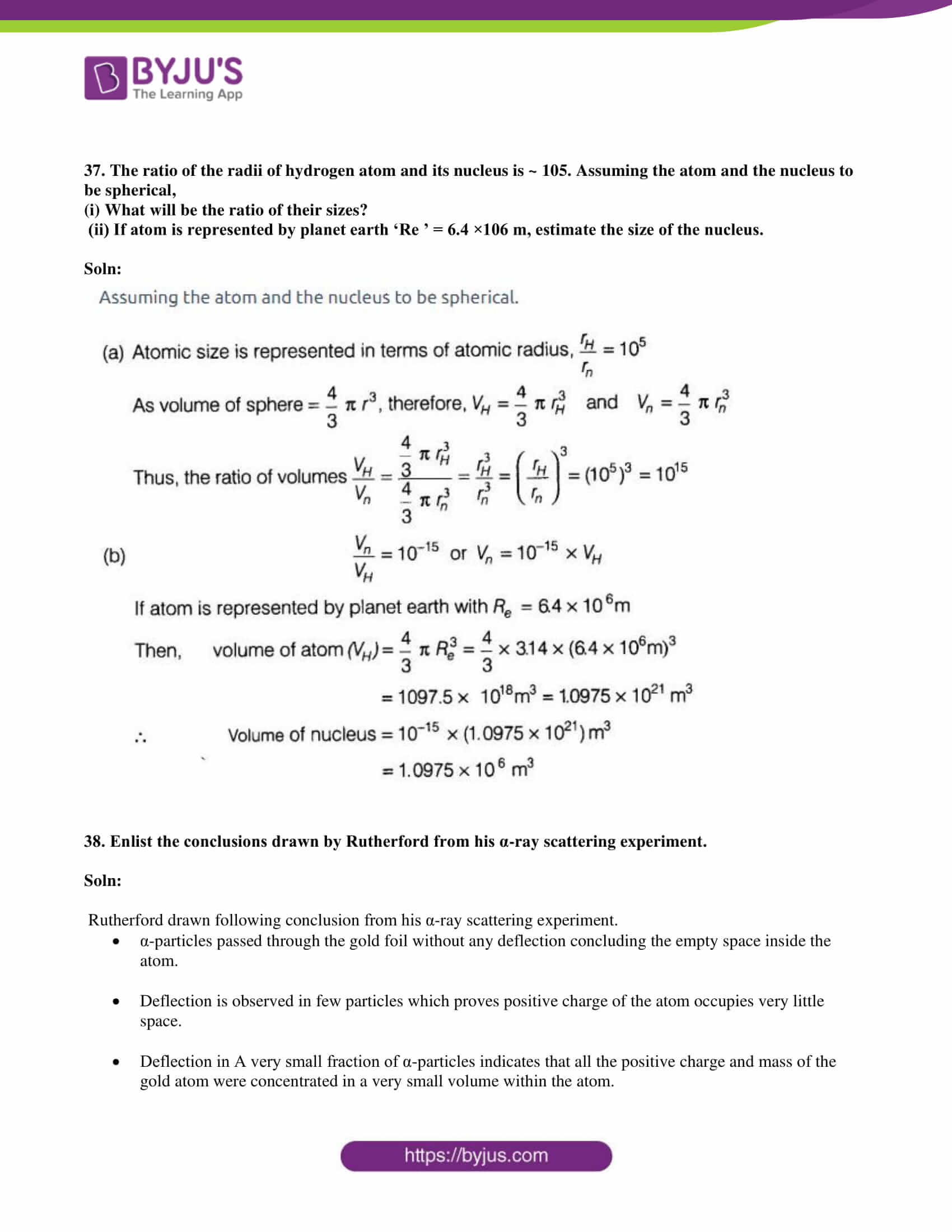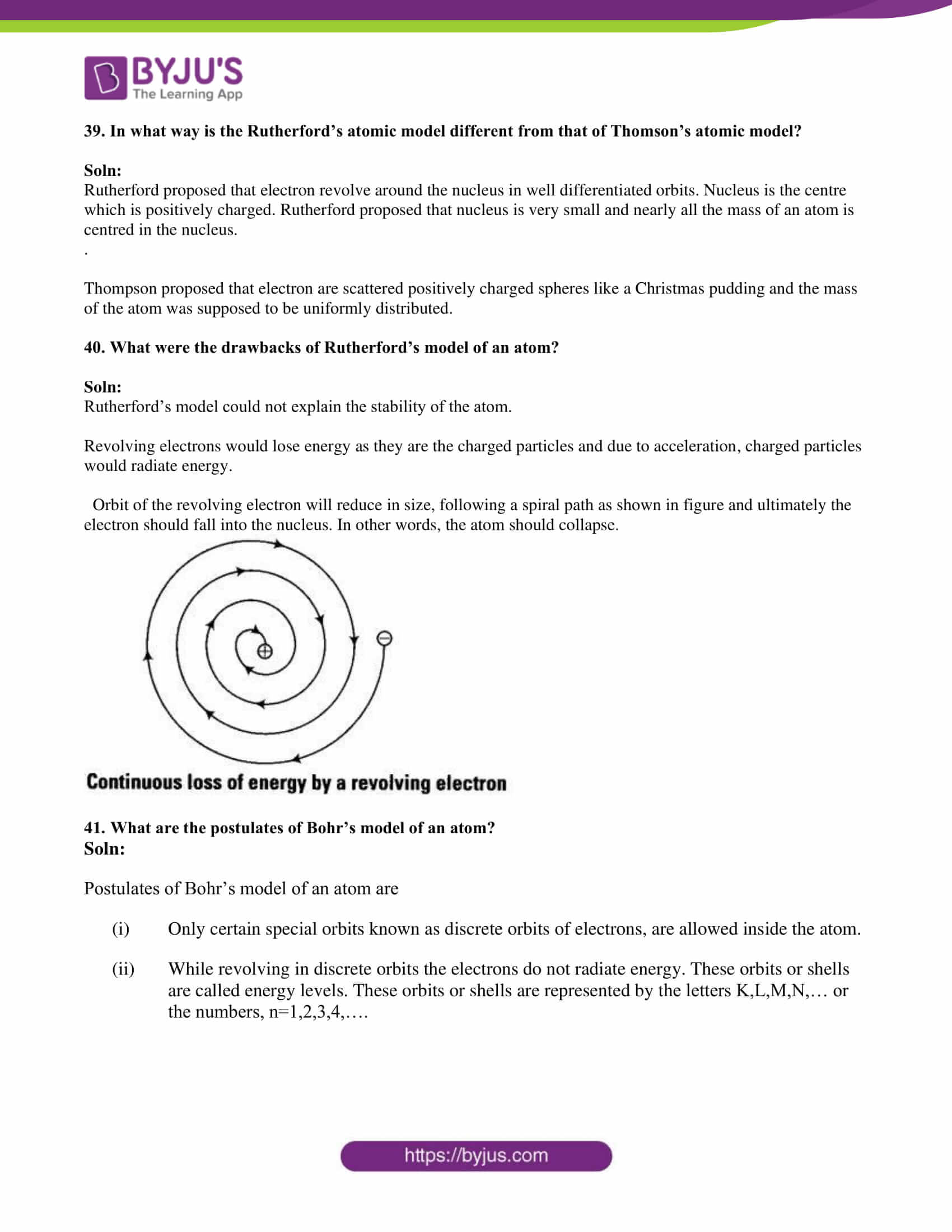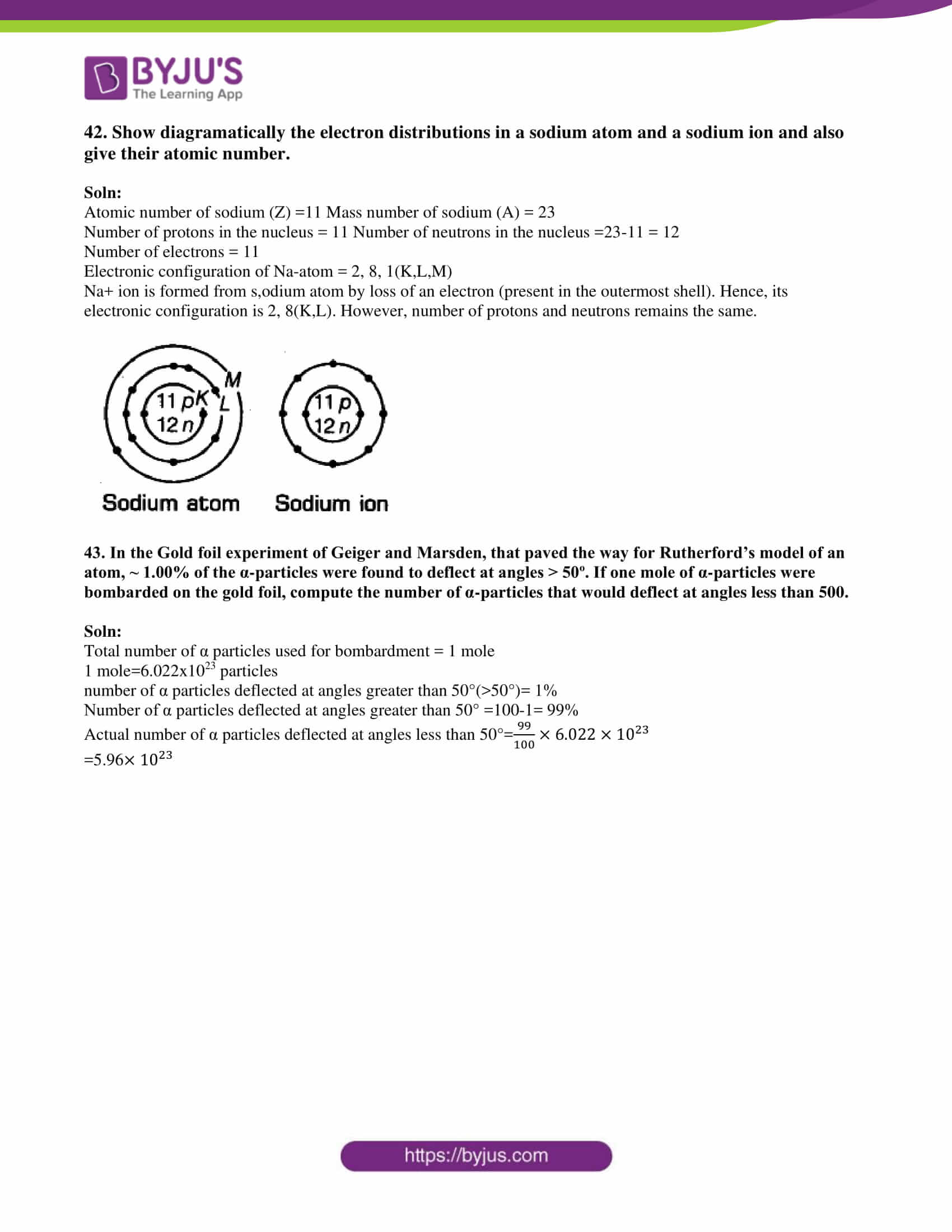### Multiple Choice Questions

1. Which of the following correctly represent the electronic distribution in the Mg atom?

(a) 3, 8, 1

(b) 2, 8, 2

(c) 1, 8, 3

(d) 8, 2, 2

Soln:

Explanation :

Atomic number of Mg is 12 hence electronic distribution will be 1s22s22p63s2.

2. Rutherford’s ‘alpha (α) particles scattering experiment’ resulted in to discovery of

(a) Electron

(b) Proton

(c) Nucleus in the atom

(d) Atomic mass

Soln:

Answer is (c) Nucleus in the atom

Explanation:

Rutherford’s ‘alpha (α) particles scattering experiment’ experiment concludes that alpha particles returned to their original path. This showed the presence of a nucleus in the centre.

3. The number of electrons in an element X is 15 and the number of neutrons is 16. Which of the following is the correct representation of the element?

a)  31X15

b) 31X16

c) 16X15

d) 15X16

Explanation:

Number of protons in an element depicts atomic number. A number of protons and electrons are equal in an element. Hence atomic number is written in subscript whereas the mass number is written in the subscript before the symbol of the element.

4. Dalton’s atomic theory successfully explained

(i) Law of conservation of mass

(ii) Law of constant composition

(iv) Law of multiple proportion

(a) (i), (ii) and (iii)

(b) (i), (iii) and (iv)

(c) (ii), (iii) and (iv)

(d) (i), (ii) and (iv)

Soln:

Answer is (d) (i), (ii) and (iv)

Explanation:

Dalton’s theory explains Law of conservation of mass, Law of constant composition, Law of multiple proportions. But it never gives any details of Law of radioactivity.

5. Which of the following statements about Rutherford’s model of atom are correct?

(i) considered the nucleus as positively charged

(ii) established that the α–particles are four times as heavy as a hydrogen atom

(iii) can be compared to solar system

(iv) was in agreement with Thomson’s model

(a) (i) and (iii)

(b) (ii) and (iii)

(c) (i) and (iv)

(d) only (i)

Soln:

Answer is (a) (i) and (iii)

Explanation:

Positively charged alpha particles were deflected by the nucleus. This shows the nucleus is positively charged. Rutherford also postulated that electrons are arranged in an atom around the nucleus like planets arranged around the sun.

6. Which of the following are true for an element?

(i) Atomic number = number of protons + number of electrons

(ii) Mass number = number of protons + number of neutrons

(iii) Atomic mass = number of protons = number of neutrons

(iv) Atomic number = number of protons = number of electrons

(a) (i) and (ii)

(b) (i) and (iii)

(c) (ii) and (iii)

(d) (ii) and (iv)

Solution:

Answer is (d) (ii) and (iv)

Explanation:

Atomic number Z is the number of protons present in an electron which is also equal to a number of the electron in an atom. Since the mass of a neutron is negligible, a number of protons and electron is added to obtain a mass number of an element.

7. In the Thomson’s model of atom, which of the following statements are correct?

(i) the mass of the atom is assumed to be uniformly distributed over the atom

(ii) the positive charge is assumed to be uniformly distributed over the atom

(iii) the electrons are uniformly distributed in the positively charged sphere

(iv) the electrons attract each other to stabilise the atom

(a) (i), (ii) and (iii)

(b) (i) and (iii)

(c) (i) and (iv)

(d) (i), (iii) and (iv)

Soln:

Answer is(a) (i), (ii) and (iii)

Explanation:

According to Thomson, the model of an atom is similar to a Christmas pudding.The electrons are studded like currants in a positively charged sphere like Christmas pudding and the mass of the atom was supposed to be uniformly distributed.

8. Rutherford’s α–particle scattering experiment showed that

(i) electrons have a negative charge

(ii) the mass and positive charge of the atom is concentrated in the nucleus

(iii) neutron exists in the nucleus

(iv) most of the space in the atom is empty Which of the above statements are correct?

(a) (i) and (iii)

(b) (ii) and (iv)

(c) (i) and (iv)

(d) (iii) and (iv

Soln:

Answer is (b) (ii) and (iv)

Explanation:

An atom consists of a positively charged, dense and very small nucleus which have all the protons and neutrons. The positive charge is due to protons, as neutrons have no charge. Here the space is empty because alpha particles pass straight through the gold foil without any deflection.

Thomson explained that electron s have a negative charge. Existence of neutron was discovered by Chadwick.

9. The ion of an element has 3 positive charges. Mass number of the atom is 27 and the number of neutrons is 14. What is the number of electrons in the ion?

(a) 13

(b) 10

(c) 14

(d) 16

Soln:

Explanation:

Mass number (A) of the atom = 27

Number of the neutron in the atom =14

Number of Electrons=Mass number-Number of neutrons=27-14

Number of electrons= 13

Since ions of the element have 3 positive charges number of the electron in the ion is 13-3 which equal 10.

10. Identify the Mg2+ ion from the Fig.4.1 where, n and p represent the number of neutrons and protons respectively.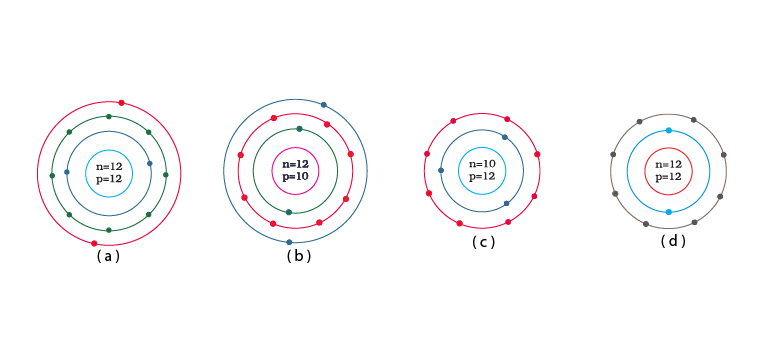Soln:

Explanation:

Number of protons in Mg atom = 2+ 8 + 2 = 12

Number of neutrons in Mg atom = 24 -12 = 12

[as mass number of Mg atom = 24 and number of neutrons = mass number – number of protons]

Therefore, option (d) is the correct answer

11. In a sample of ethyl ethanoate (CH3COOC2H5) the two oxygen atoms have the same number of electrons but different number of neutrons. Which of the following is the correct reason for it?

(a) One of the oxygen atoms has gained electrons

(b) One of the oxygen atoms has gained two neutrons

(c) The two oxygen atoms are isotopes

(d) The two oxygen atoms are isobars.

Soln:

Answer is(c) The two oxygen atoms are isotopes

Explanation:

Two Oxygen atoms in CH3COOC2H5 can have a different number of neutrons only if the two O-atoms are isotopes. Isotopes of an element have the same number of protons (and electrons) but a different number of neutrons.

12. Elements with valency 1 are

(a) always metals

(b) always metalloids

(c) either metals or non-metals

(d) always non-metals

Soln:

Answer is (c) either metals or non-metals.

Explanation:

If the element shows positive valency it is a metal and if the element shows negative valency it will be a non-metal.

13. The first model of an atom was given by

(a) N. Bohr

(b) E. Goldstein

(c) Rutherford

(d) J.J. Thomson

Soln:

14. An atom with 3 protons and 4 neutrons will have a valency of

(a) 3

(b) 7

(c) 1

(d) 4

Soln:

15. The electron distribution in an aluminium atom is

(a) 2, 8, 3

(b) 2, 8, 2

(c) 8, 2, 3

(d) 2, 3, 8

Soln:

Answer is (a) 2, 8, 3

Explanation:

Atomic number of Aluminium is 13, the First shell can have a maximum of 2 electron and the second shell holds a maximum of 8 electrons. Hence option a is the right answer.

16. Which of the following in Fig. 4.2 do not represent Bohr’s model of an atom correctly?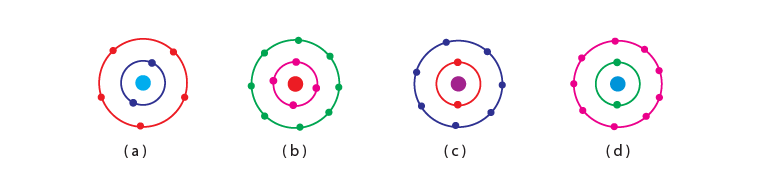(a) (i) and (ii)

(b) (ii) and (iii)

(c) (ii) and (iv)

(d) (i) and (iv)

Soln:

Explanation :

The first shell can have a maximum of 2 electron and the second shell can have a maximum of 8 electrons hence ii and iv do not represent Bohr’s model of an atom correctly.

17. Which of the following statement is always correct?

(a) An atom has equal number of electrons and protons.

(b) An atom has equal number of electrons and neutrons.

(c) An atom has equal number of protons and neutrons.

(d) An atom has equal number of electrons, protons and neutrons.

Soln:

Answer is (a) An atom has equal number of electrons and protons.

Explanation:

An atom is electrically neutral because the number of protons is always equal to a number of electrons. Hence option a) is right.

18. Atomic models have been improved over the years. Arrange the following atomic models in the order of their chronological order

(i) Rutherford’s atomic model

(ii) Thomson’s atomic model

(iii) Bohr’s atomic model

(a) (i), (ii) and (iii)

(b) (ii), (iii) and (i)

(c) (ii), (i) and (iii)

(d) (iii), (ii) and (i)

Soln:

Answer is (c) (ii), (i) and (iii)

Explanation:

Thomson’s atomic model was proposed in the year 1904

Rutherford’s atomic model was proposed in the year 1911

Bohr’s atomic model was proposed in the year 1913

19. Is it possible for the atom of an element to have one electron, one proton and no neutron. If so, name the element.

Soln:

Yes, Hydrogen is the element which is having only 1 proton and 1 electron and no neutron hence there is no repulsive force in the nucleus hence it is stable.

20. Write any two observations which support the fact that atoms are divisible

• Ionic compounds are formed because of the formation of ions that involves the transfer of electrons.
• The presence of isotopes for the same element is possible due to the difference in the number of neutrons.

Soln:

35Cl and 37Cl cannot have different valencies because they are the isotopes of the same element.

22. Why did Rutherford select a gold foil in his α–ray scattering experiment?

Soln:

Rutherford used gold for his scattering experiment because gold is the most malleable metal and he wanted the thinnest layer as possible.

Therefore, Rutherford selected a Gold foil in his alpha scattering experiment.

23. Find out the valency of the atoms represented by the Fig. 4.3 (a) and (b).Soln:

Atom (a) has zero valencies as it has 8 electrons in its valence shell making the configuration stable.

Atom (b) has a valency of +1 as it has 7 electrons in its outermost shell. It can accept 1 electron to achieve octet configuration.

24. One electron is present in the outer most shell of the atom of an element X. What would be the nature and value of charge on the ion formed if this electron is removed from the outer most shell?

Soln:

If an electron is removed from the outermost shell a cation will be formed and the charge of the element will be +1.

25. Write down the electron distribution of chlorine atom. How many electrons are there in the L shell? (Atomic number of chlorine is 17).

Soln:

Atomic number of chlorine atom = 17

So, its electronic configuration is

K L M

2 8 7

L shell of chlorine contains 8 electrons.

26. In the atom of an element X, 6 electrons are present in the outermost shell. If it acquires noble gas configuration by accepting requisite number of electrons, then what would be the charge on the ion so formed?

Soln:

In this atom, 6 electrons are already present in its outermost orbitals. In order to attain a noble gas configuration element has to accept two-electron hence its charge is -2.

27. What information do you get from the Fig. 4.4 about the atomic number, mass number and valency of atoms X, Y and Z? Give your answer in a tabular form.Soln

Atomic number, mass number and valency of atoms X, Y and Z

 Atomic no Mass no Valency X 5 11 3 Y 8 18 2 Z 15 31 3.5

28. In response to a question, a student stated that in an atom, the number of protons is greater than the number of neutrons, which in turn is greater than the number of electrons. Do you agree with the statement? Justify your answer.

Soln

The statement is wrong because the number of protons can never be greater than the number of neutrons. The number of protons will always be less than or equal to the number of neutrons. The number of electrons and protons are always equal in a neutral atom.

29. Calculate the number of neutrons present in the nucleus of an element X which is represented as 31X15

Soln:

Mass number = No. of protons + No. of neutrons = 31

∴ Number of neutrons = 31– number of protons

= 31–15

= 16

30. Match the names of the Scientists given in column A with their contributions towards the understanding of the atomic structure as given in column B

(A) – (B)

(a) Ernest Rutherford – (i) Indivisibility of atoms

(b) J.J.Thomson – (ii) Stationary orbits

(c) Dalton – (iii) Concept of the nucleus

(d) Neils Bohr – (iv) Discovery of electrons

(e) James Chadwick -(v) Atomic number

(f) E. Goldstein – (vi) Neutron

(g) Mosley – (vii) Canal rays

Soln:

(A) – (B)

(a) Ernest Rutherford – (iii) Concept of the nucleus

(b) J.J.Thomson – (iv) Discovery of electrons

(c) Dalton – (i) Indivisibility of atoms

(d) Neils Bohr – (ii) Stationary orbits

(e) James Chadwick – (vi) Neutron

(f) E. Goldstein – (vii) Canal rays

(g) Mosley – (v) Atomic number

31. The atomic number of calcium and argon are 20 and 18 respectively, but the mass number of both these elements is 40. What is the name given to such a pair of elements?

Soln:

Elements with different atomic numbers but same mass numbers are known as isobars. Calcium and argon are isobars.

32. Complete Table 4.1 on the basis of information available in the symbols given below

(a) 35 17 Cl (b) 12 6 C (c) 81 35 Br

 Element np nn

Soln:

 Element np nn Cl 17 18 C 6 6 Br 35 46

33. Helium atom has 2 electrons in its valence shell but its valency is not 2, Explain

Soln:

Helium has 2 electrons in its outermost shell thereby completing duplet configuration. Hence it has no valence shell left empty making its valency 0.

34. Fill in the blanks in the following statements

(a) Rutherford’s α-particle scattering experiment led to the discovery of the ———

(b) Isotopes have same ———but different———.

(c) Neon and chlorine have atomic numbers 10 and 17, respectively. Their valencies will be———and———respectively.

(d) The electronic configuration of silicon is ———and that of sulphur is ———

Soln:
a) Rutherford’s α-particle scattering experiment led to the discovery of the atomic nucleus
b) Isotopes have same atomic number but different mass number
c) Neon and chlorine have atomic numbers 10 and 17, respectively. Their valencies will be 0 and 1, respectively.
d) The electronic configuration of silicon is 2.8.4 and that of sulphur is 2.8.6

35. An element X has a mass number 4 and atomic number 2. Write the valency of this element?

Soln:

Mass number = 4

Atomic number = 2

X is Helium.

It has 0 valency and it will not react with any other atom because it has its outer shell filled.

36. Why do Helium, Neon and Argon have a zero valency?

Soln:

Helium has 2 electrons in its outermost orbit, thus filling shell 1 and forming duplet configuration in the valence shell. Neon has 8 electrons in their valence orbit hence completing duplet configuration. In the same way, Argon and Neon have 8 electrons in its outermost shelling completing octet configuration. As these elements have a maximum electron in their valence shell thus reach stable electron configuration and they will not take part in any sort of chemical reactions.

37. The ratio of the radii of hydrogen atom and its nucleus is ~ 105. Assuming the atom and the nucleus to be spherical,

(i) What will be the ratio of their sizes?

(ii) If atom is represented by planet earth ‘Re ’ = 6.4 ×106 m, estimate the size of the nucleus.

Soln: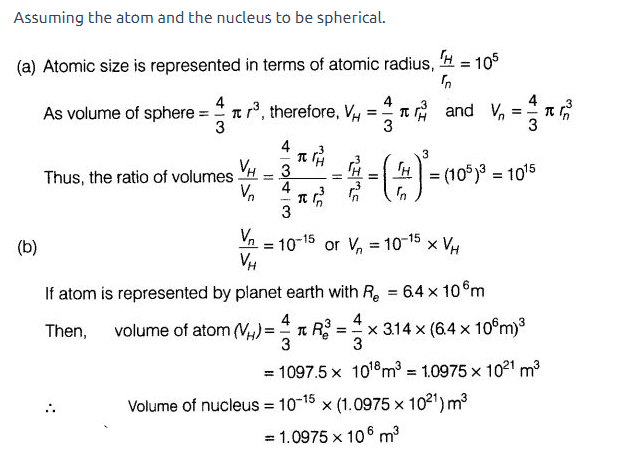38. Enlist the conclusions drawn by Rutherford from his α-ray scattering experiment.

Soln:

Rutherford drawn following conclusion from his α-ray scattering experiment.

• α-particles passed through the gold foil without any deflection concluding the empty space inside the atom.
• Deflection is observed in few particles which prove the positive charge of the atom occupies very little space.
• Deflection in A very small fraction of α-particles indicates that all the positive charge and mass of the gold atom were concentrated in a very small volume within the atom.

39. In what way is the Rutherford’s atomic model different from that of Thomson’s atomic model?

Soln:

Rutherford proposed that electron revolves around the nucleus in well-differentiated orbits. The nucleus is the centre which is positively charged. Rutherford proposed that the nucleus is very small and nearly all the mass of an atom is centred in the nucleus.

.

Thompson proposed that electron are scattered positively charged spheres like a Christmas pudding and the mass of the atom was supposed to be uniformly distributed.

40. What were the drawbacks of Rutherford’s model of an atom?

Soln:

Rutherford’s model could not explain the stability of the atom.

Revolving electrons would lose energy as they are the charged particles and due to acceleration, charged particles would radiate energy.

The orbit of the revolving electron will reduce in size, following a spiral path as shown in the figure and ultimately the electron should fall into the nucleus. In other words, the atom should collapse.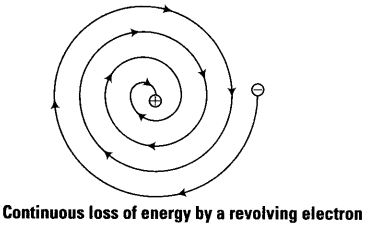41. What are the postulates of Bohr’s model of an atom?

Soln:

Postulates of Bohr’s model of an atom are

1. Only certain special orbits known as discrete orbits of electrons are allowed inside the atom.
2. While revolving in discrete orbits the electrons do not radiate energy. These orbits or shells are called energy levels. These orbits or shells are represented by the letters K, L, M, N,… or the numbers, n=1,2,3,4,….

42. Show diagrammatically the electron distributions in a sodium atom and a sodium ion and also give their atomic number.

Soln:

Atomic number of sodium (Z) =11 Mass number of sodium (A) = 23

Number of protons in the nucleus = 11 Number of neutrons in the nucleus =23-11 = 12

Number of electrons = 11

Electronic configuration of Na-atom = 2, 8, 1(K,L,M)

Na+ ion is formed from sodium atom by loss of an electron (present in the outermost shell). Hence,

the electronic configuration is 2, 8(K, L). However, the number of protons and neutrons remains the same.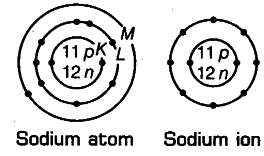43. In the Gold foil experiment of Geiger and Marsden, that paved the way for Rutherford’s model of an atom, ~ 1.00% of the α-particles were found to deflect at angles > 50º. If one mole of α-particles were bombarded on the gold foil, compute the number of α-particles that would deflect at angles less than 500.

Soln:

Total number of α particles used for bombardment = 1 mole

1 mole=6.022×1023 particles

number of α particles deflected at angles greater than 50°(>50°)= 1%

Number of α particles deflected at angles greater than 50° = 100-1 = 99%

The actual number of α particles deflected at angles less than 50°= 99/100 × 6.022 × 1023

=5.96 × 1023

### NCERT Exemplar for Chapter 4 Structure of the Atom

Before solving the questions related to the chapter Structure of the Atom, let us learn what an atom is. An atom is the smallest constituent unit of ordinary matter that has the properties of chemical elements. Important topics related to this chapter are as listed.

• Atomic Number
• Mass Number
• Isotopes
• Isobars

Important Topics of the NCERT Exemplar for Chapter 4 Structure of the Atom

1. Charged Particles in Matter
2. The Structure of An atom (2a) Thomson’s Model of an Atom (2b) Rutherford’s Model of an Atom (2c) Bohr’s Model of Atom (2d) Neutrons
3. How Are Electrons Distributed in Different Orbits (Shells)
4. Valency
5. Atomic Number and Mass Number (5a) Atomic Number (5b) Mass Number
6. Isotopes (6a) Isobars

BYJU’S has the country’s top teachers and subject experts as mentors. Their experience will help you immensely in understanding the concepts and keeping them memorised for a long time. For NCERT study materials and to know about our innovative teaching methodology, download BYJU’S – The Learning App.

## Frequently Asked Questions on NCERT Exemplar Solutions for Class 9 Science Chapter 4

Q1

### What are the types of questions present in Chapter 4 of NCERT Exemplar Solutions for Class 9 Science?

The types of questions present in Chapter 4 of NCERT Exemplar Solutions for Class 9 Science are as follows:
1. Multiple-choice questions – 18 questions
2. Short answers – 16 questions
3. Long answers – 8 questions
Q2

### List out the topics discussed in Chapter 4 of NCERT Exemplar Solutions for Class 9 Science.

The topics discussed in Chapter 4 of NCERT Exemplar Solutions for Class 9 Science are as follows:
1. Charged Particles in Matter
2. The Structure of an Atom
(2a) Thomson’s Model of an Atom
(2b) Rutherford’s Model of an Atom
(2c) Bohr’s Model of Atom
(2d) Neutrons
3. How Are Electrons Distributed in Different Orbits (Shells)
4. Valency
5. Atomic Number and Mass Number
(5a) Atomic Number
(5b) Mass Number
6. Isotopes
(6a) Isobars
Q3

### Where can I get NCERT Exemplar Solutions for Class 9 Science Chapter 4 online?

Class 9 is an important stage in every student’s life. The marks obtained in Class 9 will help decide the career path of students. For this purpose, the faculty at BYJU’S has created solutions for all chapters based on the CBSE syllabus. Students can download NCERT Solutions available in PDF format from BYJU’S. The solutions are framed keeping in mind the exam preparation of students, irrespective of their understanding abilities.